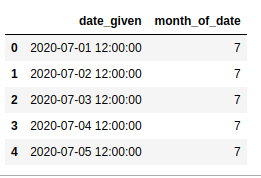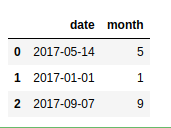Related Articles
Get Month from date in Pandas – Python
• Last Updated : 02 Jul, 2020

Pandas has many inbuilt methods that can be used to extract the month from a given date that are being generated randomly using the random function or by using Timestamp function or that are transformed to date format using the to_datetimefunction. Let’s see few examples for better understanding.

Example 1

 `import` `pandas as pd`` ` ` ` `dti ``=` `pd.date_range(``'2020-07-01'``, periods ``=` `4``, freq ``=``'M'``)``print``(dti.month)`
Output:
```Int64Index([7, 8, 9, 10], dtype='int64')
```

Example 2

 `import` `pandas as pd``import` `numpy as np``import` `datetime`` ` `date1 ``=` `pd.Series(pd.date_range(``'2020-7-1 12:00:00'``, periods ``=` `5``))``df ``=` `pd.DataFrame(``dict``(date_given ``=` `date1))`` ` `df[``'month_of_date'``] ``=` `df[``'date_given'``].dt.month``df`
Output:Example 3

 `import` `pandas as pd``import` `datetime``import` `numpy as np`` ` ` ` `dates ``=``[``'14 / 05 / 2017'``, ``'2017'``, ``'07 / 09 / 2017'``]`` ` `frame ``=` `pd.to_datetime(dates, dayfirst ``=` `True``)``frame ``=` `pd.DataFrame([frame]).transpose()``frame[``'date'``]``=` `frame``frame[``'month'``]``=` `frame[``'date'``].dt.month``frame.drop(``0``, axis ``=` `1``, inplace ``=` `True``)`` ` `frame`
Output:Attention geek! Strengthen your foundations with the Python Programming Foundation Course and learn the basics.

To begin with, your interview preparations Enhance your Data Structures concepts with the Python DS Course. And to begin with your Machine Learning Journey, join the Machine Learning – Basic Level Course

My Personal Notes arrow_drop_up